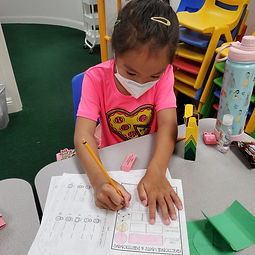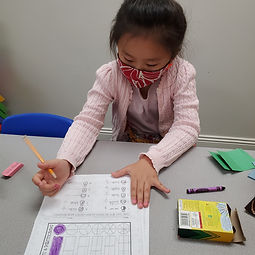## Ms. Raejean

### Target 1​

###### Lesson Type:

Continuation

Number Operation

:

Fractional Numbers

Visually distinguish equal parts of the same whole.

###### 1:

Distinguish between equal and unequal parts.

###### 2:

Describe if parts are the same or if one is bigger or smaller.

2nd

###### Vocabulary:

Fractions, Equal, Unequal, Halves, Thirds, Fourths, Fifths, Sixths, Eighths

Activities:

Students used pattern blocks to explore equal and unequal parts. They covered hexagons with 6 triangles, 3 rhombuses, and 2 trapezoids. We identifed the equal parts as halves, thirds, and sixths. Students also covered hexagons with a combination of pattern blocks - 1 trapezoid and 3 triangles or 2 rhombuses and 2 triangles, etc. We identified the unequal parts and discussed the bigger versus smaller parts. Then students identified the shapes divided into equal parts with a checkmark, and shapes divided into unequal parts were marked with an X (on a worksheet). Students marked the bigger parts with a smiley face. Finally, students divided wholes (rectangles and circles) into equal parts and wrote the names of the fractional parts and fraction (ex. halves 1/2, thirds 1/3). We discussed how the top number tells how many equal parts are counted, and the bottom number tells how many equal parts are in the whole.### Home Exploration

###### Guiding Questions:## Absent Students:

### Target 2

:

###### 1:

Understand congruence is a geometric word that indicates something is the same shape and size.

2nd

###### Vocabulary:

Congruent, Similar

Activities:

We reviewed congruent - same shape, same size. Students identified congruent shapes in a given set of shapes. We also talked about similar shapes - same shape, but different size. We examined how congruent shapes can be turned or "crooked", but they are still the same shape and same size.### Home Exploration

###### Guiding Questions:### Target 3

:

###### Vocabulary:

Activities:### Home Exploration